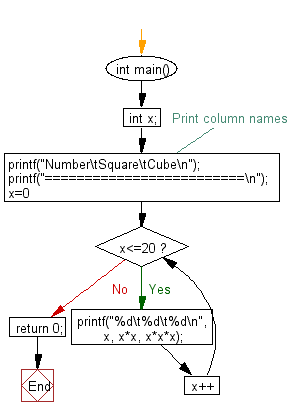﻿ C : Calculate the squares and cubes of the numbers 0-20

# C Exercises: Calculate and prints the squares and cubes of the numbers from 0 to 20

## C Basic Declarations and Expressions: Exercise-76 with Solution

Write a C program to calculate and print the squares and cubes of the numbers from 0 to 20. It uses tabs to display them in a table of values.

Sample Solution:

C Code:

``````#include<stdio.h>
int main()
{
int x;

/* Print column names */
printf("Number\tSquare\tCube\n");
printf("=========================\n");

// Loop to calculate and print squares and cubes
for(x=0; x<=20; x++)
printf("%d\t%d\t%d\n", x, x*x, x*x*x);

return 0;
}
``````

Sample Output:

```Number	Square	Cube
=========================
0	0	0
1	1	1
2	4	8
3	9	27
4	16	64
5	25	125
6	36	216
7	49	343
8	64	512
9	81	729
10	100	1000
11	121	1331
12	144	1728
13	169	2197
14	196	2744
15	225	3375
16	256	4096
17	289	4913
18	324	5832
19	361	6859
20	400	8000
```

Pictorial Presentation:Flowchart:C programming Code Editor:

What is the difficulty level of this exercise?

Test your Programming skills with w3resource's quiz.

﻿